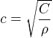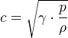# Glossary of Aviation Terms | Speed of Sound

## Speed of Sound | Paramount Business Jets

The speed of sound is 761 mph, 1225 km/h, 340 m/s, or 661 knots at the standard temperature, which is 15 degrees Celsius. Unlike the speed of light, which remains relatively constant almost wherever it goes, the speed of sound changes depending on the density and temperature of what it is travelling through. If the temperature is colder, the speed of sound travels slower, and if the temperature is hotter, the speed of sound travels faster. This is because the air molecules have more energy at hotter temperatures and the molecules travel at a faster rate, which in turn helps the sound waves further accelerate. The speed of sound is a vibration, and the more it has to vibrate off of, the more it will be able to resonate and keep moving. For example, the speed of sound will travel faster underwater than in the air because the vibration it gives off has more to repel itself off of.

The speed of sound also affects the aircraft's forces. If the aircraft is moving slower than the speed of sound, then it is said that the aircraft is subsonic. If the aircraft is moving close to or a little more than the speed of sound, then the aircraft is transonic. If the aircraft is moving faster than the speed of sound, then the aircraft is supersonic. The aircraft's speed in relation to the speed of sound is called the Mach number. The Mach number is expressed as a percentage of the speed of sound. For example, if an aircraft was moving at 600 knots, then the aircraft's Mach number would be 0.9 the speed of sound. Depending on the shape and size of the aircraft and the atmospheric conditions present, shockwaves can form from the aircraft moving close to or faster than the speed of sound. A shock wave is the compression of the air due to the speed of the aircraft through the air. These can be either negative or positive for the aircraft, but more often than not they are negative.

The speed of sound is mathematically defined as the letter “c”. As an equation, it is generally represented by:where: c = the speed of sound, C = coefficient of stiffness, g = density

Otherwise, when dealing with the speed of sound traveling through a gas, the following equation is more commonly used:where: c = the speed of sound, y = adiabatic index, P = pressure, g = density

## Get a Quote for Your trip

•••••••••